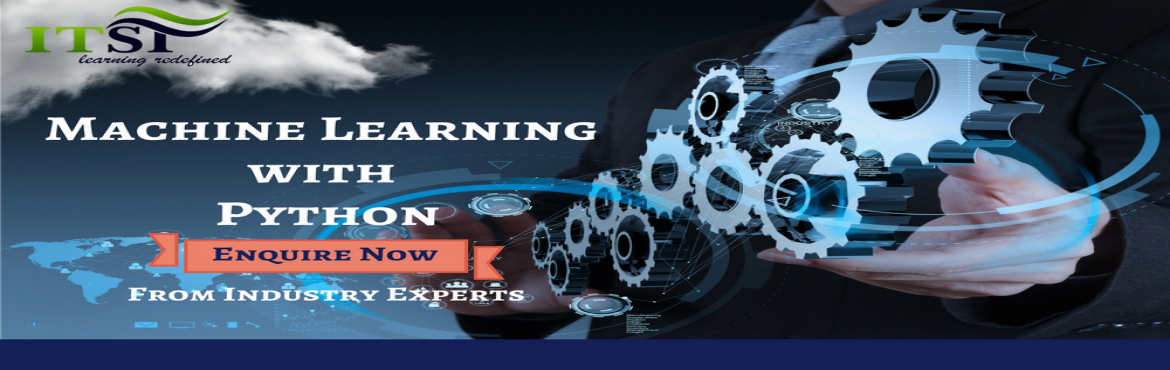# Machine Learning With Python

• ## Demo On Machine Learning

Sale Date Ended

0
Sold Out

#### Invite friends

Page Views : 15

Chapter 1: Data Structures
Python Basics Loops & Built in Functions
User defined Functions

Chapter 2 : Indexing in Python
Python Data Manipulation Outlier Detection
Missing value Imputation

Chapter 3: Measure of Central Tendency
Basic Statistics Histograms, Boxplots,
Frequency Distributions

Chapter 4: Central Limit Theorem
Advanced Statistics Hypothesis Testing, Binomial Theorem, Z test, T test, Chi Square Test

Chapter 5: Simple Linear Regression

Linear Regression
Assumptions of Linear Regression

Evaluating Accuracy of Regression Models
Multivariate Linear Regression

Chapter 6 Linear Regression hands on in Python with Case Study
Linear Regression- Case Study

Chapter 7 : Logistic response Function, Logit, Odds, Evaluating Accuracy of Classification Models, ROC curve, AUC
Logistic Regression

Chapter 8: Logistic Regression hands on in Python with Case Study
Logistic Regression- Case Study

Chapter 9 : Decision Trees, Gini Index
Decision Trees Decision Trees for classification & Regression
Overfitting In decision Trees

Chapter 10: Random Forest, Bagging or Bootstrap Aggregating, OOB (out of bag Error), Variable Importance plot from RF
Random Forest

Chapter 11: Decision Tree & Random Forest Hands-On in Python with Case Study
Decision Tree & RF- Case Study

Chapter 12: Bias Variance Trade-off, Removing Bias & Variance in ML Models, LOOCV, K Fold Cross Validation
Bias vs Variance; Cross validation

Chapter 13: SVM Theory, Kernel Trick, C

Support Vector Machines
gamma Parameters

Chapter 14: Support Vector Machine hands-on in Python with Case Study
Support Vector Machines- Case Study

Chapter 15: Unsupervised Learning, Euclidean, Manhattan Distance, Dendograms, Theory
Hierarchical Clustering